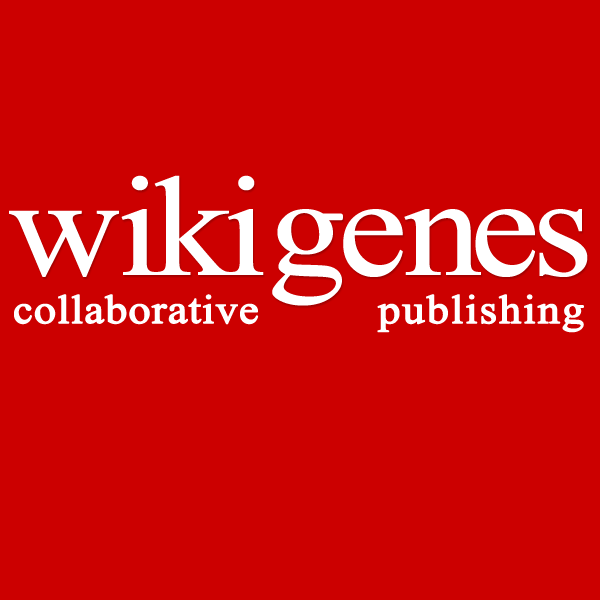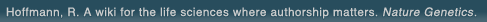# The world's first wiki where authorship really matters (Nature Genetics, 2008). Due credit and reputation for authors. Imagine a global collaborative knowledge base for original thoughts. Search thousands of articles and collaborate with scientists around the globe.

wikigene or wiki gene protein drug chemical gene disease author authorship tracking collaborative publishing evolutionary knowledge reputation system wiki2.0 global collaboration genes proteins drugs chemicals diseases compound
Hoffmann, R. A wiki for the life sciences where authorship matters. Nature Genetics (2008)# Falloff curves for the Reaction CH3 + O2 (+ M) --> CH3O2 (+ M) in the pressure range 2-1000 bar and the temperature range 300-700 K.

The reaction CH(3) + O(2) (+M) --> CH(3)O(2) (+M) was studied in the bath gases Ar and N(2) in a high-temperature/high-pressure flow cell at pressures ranging from 2 to 1000 bar and at temperatures between 300 and 700 K. Methyl radicals were generated by laser flash photolysis of azomethane or acetone. Methylperoxy radicals were monitored by UV absorption at 240 nm. The falloff curves of the rate constants are represented by the simplified expression k/k(infinity) approximately [x/(1 + x)]F(cent)(1/{1+[(log)(x)/)(N)(]2}) with x = k(0)/k(infinity) F(cent) approximately 0.33, and N approximately 1.47, where k(0) and k(infinity) denote the limiting low and high-pressure rate constants, respectively. At low temperatures, 300-400 K, and pressures >300 bar, a fairly abrupt increase of the rate constants beyond the values given by the falloff expressions was observed. This effect is attributed to a contribution from the radical complex mechanism as was also observed in other recombination reactions of larger radicals. Equal limiting low-pressure rate constants k(0) = [M]7 x 10(-31)(T/300 K)(-3.0) cm(6) molecule(-2) s(-1) were fitted for M = Ar and N(2) whereas limiting high-pressure rate constants k(infinity) = 2.2 x 10(-12)(T/300 K)(0.9) cm(3) molecule(-1) s(-1) were approached. These values are discussed in terms of unimolecular rate theory. It is concluded that a theoretical interpretation of the derived rate constants has to be postponed until better information of the potential energy surface is available. Preliminary theoretical evaluation suggests that there is an "anisotropy bottleneck" in the otherwise barrierless interaction potential between CH(3) and O(2).

## References

1. Falloff curves for the Reaction CH3 + O2 (+ M) --&gt; CH3O2 (+ M) in the pressure range 2-1000 bar and the temperature range 300-700 K. Fernandes, R.X., Luther, K., Troe, J. The journal of physical chemistry. A, Molecules, spectroscopy, kinetics, environment & general theory. (2006) [Pubmed]